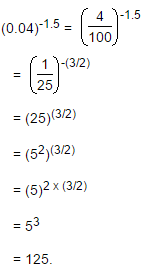Courses

# Test: Surds And Indices- 1

## 10 Questions MCQ Test Quantitative Aptitude for Banking Preparation | Test: Surds And Indices- 1

Description
This mock test of Test: Surds And Indices- 1 for Quant helps you for every Quant entrance exam. This contains 10 Multiple Choice Questions for Quant Test: Surds And Indices- 1 (mcq) to study with solutions a complete question bank. The solved questions answers in this Test: Surds And Indices- 1 quiz give you a good mix of easy questions and tough questions. Quant students definitely take this Test: Surds And Indices- 1 exercise for a better result in the exam. You can find other Test: Surds And Indices- 1 extra questions, long questions & short questions for Quant on EduRev as well by searching above.
QUESTION: 1

### Practice Quiz or MCQ (Multiple Choice Questions) with solutions are available for Practice, which would help you prepare for "Surds and Indices" under Quantitative Aptitude. You can practice these practice quizzes as per your speed and improvise the topic. The same topic is covered under various competitive examinations like - CAT, GMAT, Bank PO, SSC and other competitive examinations.   (17)3.5 x (17)? = 178

Solution: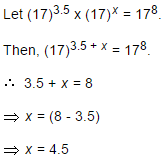QUESTION: 2

###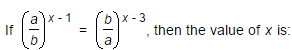Solution: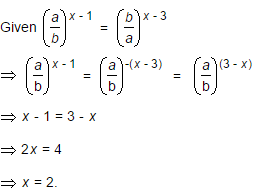QUESTION: 3

### Given that 100.48 = x, 100.70 = y and xz = y2, then the value of z is close to:

Solution: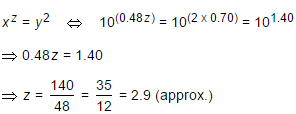QUESTION: 4

If 5a = 3125, then the value of 5(a - 3) is:

Solution: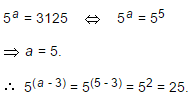QUESTION: 5

If 3(x - y) = 27 and 3(x + y) = 243, then x is equal to:

Solution:

3x - y = 27 = 33    ⇔    x - y = 3 ....(i)

3x + y = 243 = 35    ⇔    x + y = 5 ....(ii)

On solving (i) and (ii), we get x = 4.

QUESTION: 6

(256)0.16 x (256)0.09 = ?

Solution: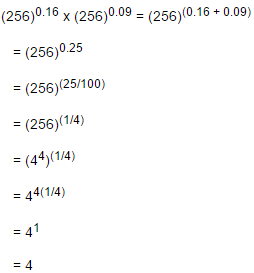QUESTION: 7

The value of [(10)150 ÷ (10)146]

Solution: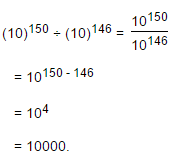QUESTION: 8Solution: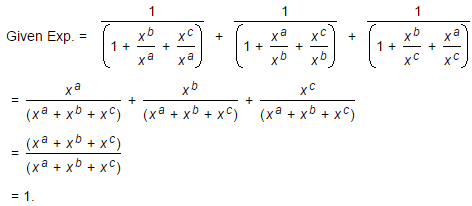QUESTION: 9

(25)7.5 x (5)2.5 ÷ (125)1.5 = 5?

Solution: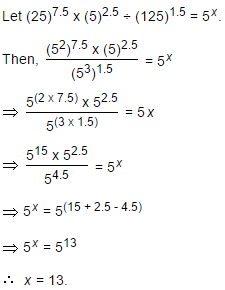QUESTION: 10

(0.04)-1.5 = ?

Solution: